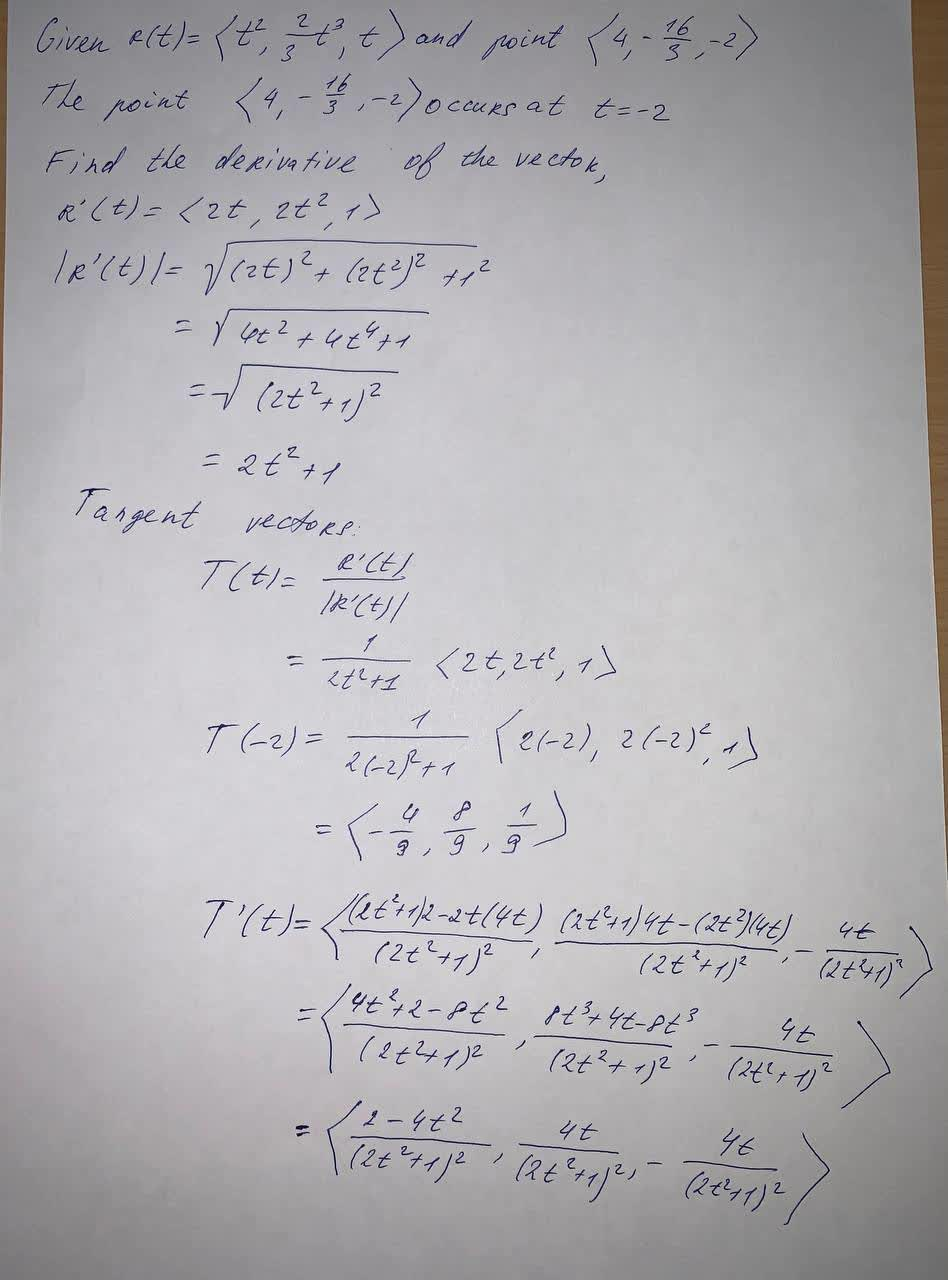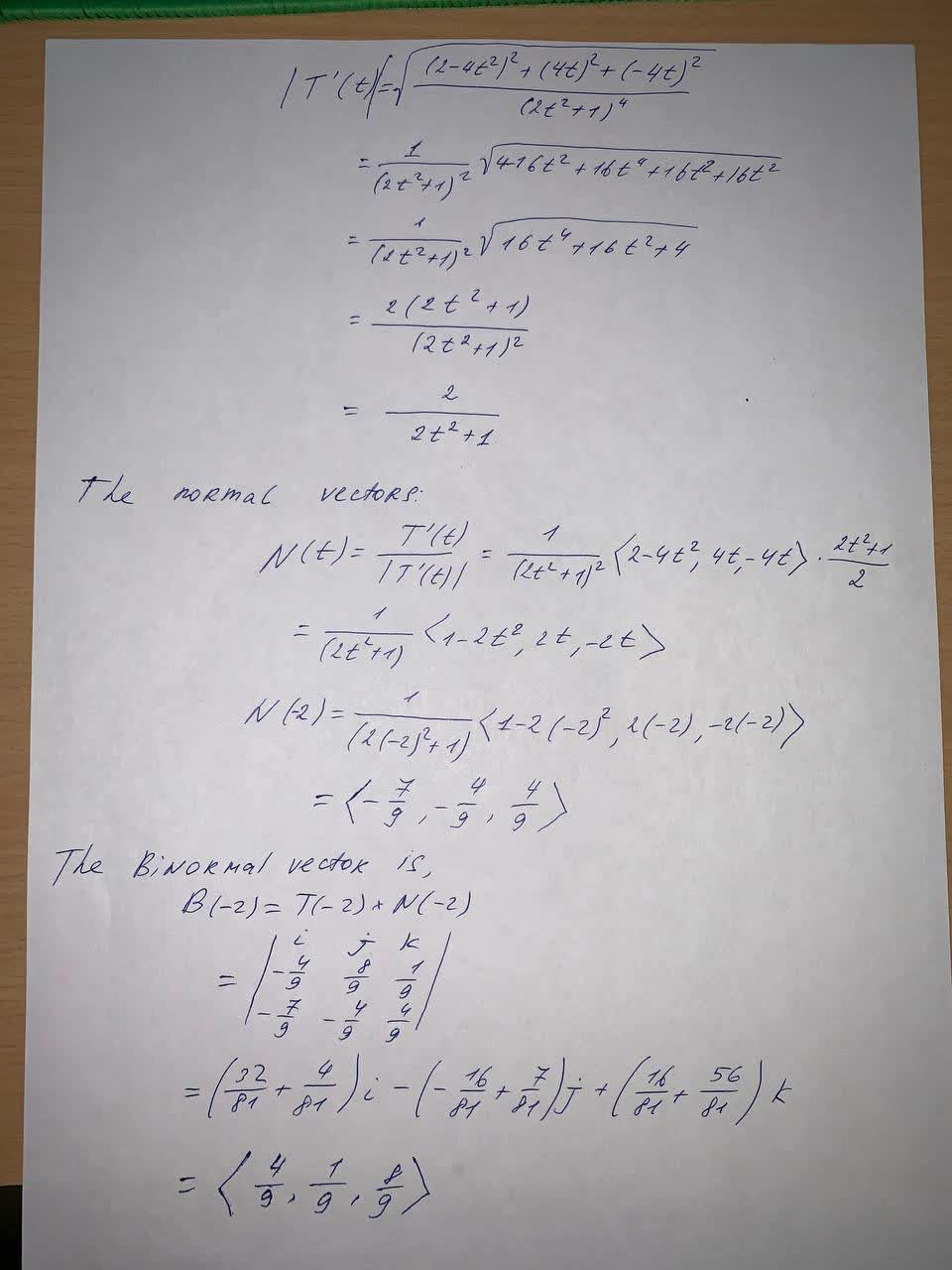# Find the vectors T, N, and B at the given point. r(t) =<t^2, \frac{2}{3}t^3 , t> and point <4,-\frac{16}{3},-2>Zoe Oneal 2021-06-01 Answered
Find the vectors T, N, and B at the given point.
$r\left(t\right)=<{t}^{2},\frac{2}{3}{t}^{3},t>$ and point $<4,-\frac{16}{3},-2>$
You can still ask an expert for help

• Questions are typically answered in as fast as 30 minutes

Solve your problem for the price of one coffee

• Math expert for every subject
• Pay only if we can solve itsmallq9
The solution to the vector is below:###### Not exactly what you’re looking for?Jeffrey Jordon

Given $R\left(t\right)=<{t}^{2},\frac{2}{3}{t}^{3},t>$ and point $<4,-\frac{16}{3},-2>$

The point $<4,-\frac{16}{3},-2>$ occursat t=-2

Find the derivative of the vector,

${R}^{\prime }\left(t\right)=<2t,2{t}^{2},1>$

$|{R}^{\prime }\left(t\right)|=\sqrt{\left(2t{\right)}^{2}+\left(2{t}^{2}{\right)}^{2}+{1}^{2}}$

$=\sqrt{4{t}^{2}+4{t}^{4}+1}$

$=\sqrt{\left(2{t}^{2}+1{\right)}^{2}}$

$=2{t}^{2}+1$

Tangent vectors:

$T\left(t\right)=\frac{{R}^{\prime }\left(t\right)}{|{R}^{\prime }\left(t\right)|}$

$=\frac{1}{2{t}^{2}+1}<2t,2{t}^{2},1>$

$T\left(-2\right)=\frac{1}{2\left(-2{\right)}^{2}+1}<2\left(-2\right),2\left(-2{\right)}^{2},1>$

$T\left(-2\right)=\frac{1}{2\left(-2{\right)}^{2}+1}<2\left(-2\right),2\left(-2{\right)}^{2},1>$

$=<-\frac{4}{9},\frac{8}{9},\frac{1}{9}>$

${T}^{\prime }\left(t\right)=<\frac{\left(2{t}^{2}+1\right)2-2t\left(4t\right)}{\left(2{t}^{2}+1{\right)}^{2}},\frac{\left(2{t}^{2}+1\right)4t-\left(2{t}^{2}\right)\left(4t\right)}{\left(2{t}^{2}+1{\right)}^{2}},-\frac{4t}{\left(2{t}^{2}+1{\right)}^{2}}>$

$=<\frac{4{t}^{2}+2-8{t}^{2}}{\left(2{t}^{2}+1{\right)}^{2}},\frac{8{t}^{3}+4t-8{t}^{3}}{\left(2{t}^{2}+1{\right)}^{2}},-\frac{4t}{\left(2{t}^{2}+1{\right)}^{2}\right)}>$

$=<\frac{2-4{t}^{2}}{\left(2{t}^{2}+1{\right)}^{2}},\frac{4t}{\left(2{t}^{2}+1{\right)}^{2}},-\frac{4t}{\left(2{t}^{2}+1{\right)}^{2}}>$

$|{T}^{\prime }\left(t\right)|=\sqrt{\frac{\left(2-4{t}^{2}{\right)}^{2}+\left(4t{\right)}^{2}+\left(-4t{\right)}^{2}}{\left(2{t}^{2}+1{\right)}^{4}}}$

$=\frac{1}{\left(2{t}^{2}+1{\right)}^{2}}\sqrt{4-16{t}^{2}+16{t}^{4}+16{t}^{2}+16{t}^{2}}$

$=\frac{1}{\left(2{t}^{2}+1{\right)}^{2}}\sqrt{16{t}^{4}+16{t}^{2}+4}$

$=\frac{2\left(2{t}^{2}+1\right)}{\left(2{t}^{2}+1{\right)}^{2}}$

$=\frac{2}{2{t}^{2}+1}$

The normal vectors.